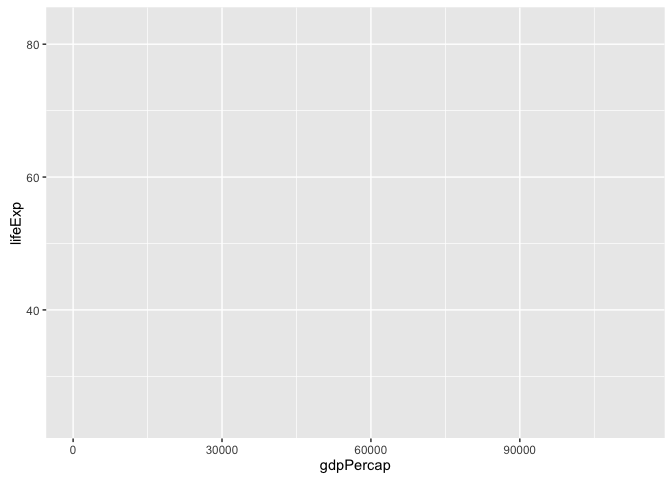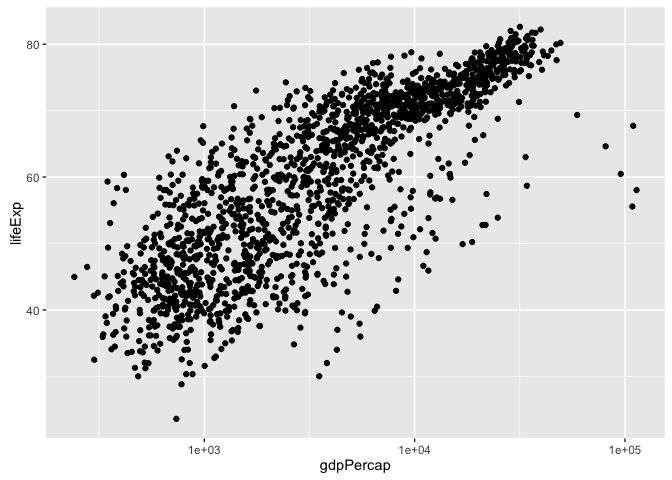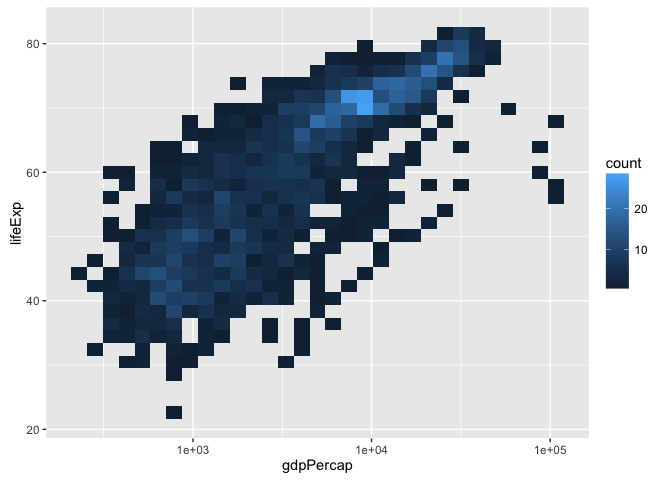# ggplot2 Tutorial

export MODULEPATH="\${MODULEPATH}:/hpc/modules/workshop"
srun -p development,htc,mic -c 1 --mem=6G --pty -t 0-2 m2_rstudio

library(tibble)
library(ggplot2)


library(gapminder)
gapminder

## # A tibble: 1,704 × 6
##        country continent  year lifeExp      pop gdpPercap
##         <fctr>    <fctr> <int>   <dbl>    <int>     <dbl>
## 1  Afghanistan      Asia  1952  28.801  8425333  779.4453
## 2  Afghanistan      Asia  1957  30.332  9240934  820.8530
## 3  Afghanistan      Asia  1962  31.997 10267083  853.1007
## 4  Afghanistan      Asia  1967  34.020 11537966  836.1971
## 5  Afghanistan      Asia  1972  36.088 13079460  739.9811
## 6  Afghanistan      Asia  1977  38.438 14880372  786.1134
## 7  Afghanistan      Asia  1982  39.854 12881816  978.0114
## 8  Afghanistan      Asia  1987  40.822 13867957  852.3959
## 9  Afghanistan      Asia  1992  41.674 16317921  649.3414
## 10 Afghanistan      Asia  1997  41.763 22227415  635.3414
## # ... with 1,694 more rows

ggplot(gapminder, aes(x = gdpPercap, y = lifeExp)) # nothing to plot yet!ggplot(gapminder, aes(x = gdpPercap, y = lifeExp)) +
geom_point()p <- ggplot(gapminder, aes(x = gdpPercap, y = lifeExp)) # just initializes


scatterplot

p + geom_point()log transformation … quick and dirty

ggplot(gapminder, aes(x = log10(gdpPercap), y = lifeExp)) +
geom_point()a better way to log transform

p + geom_point() + scale_x_log10()let’s make that stick

p <- p + scale_x_log10()


common workflow: gradually build up the plot you want re-define the object ‘p’ as you develop “keeper” commands convey continent by color: MAP continent variable to aesthetic color

p + geom_point(aes(color = continent))## add summary(p)!
plot(gapminder, aes(x = gdpPercap, y = lifeExp, color = continent)) +
geom_point() + scale_x_log10() # in full detail, up to now

## Error in plot(gapminder, aes(x = gdpPercap, y = lifeExp, color = continent)) + : non-numeric argument to binary operatoraddress overplotting: SET alpha transparency and size to a value

p + geom_point(alpha = (1/3), size = 3)add a fitted curve or line

p + geom_point() + geom_smooth()p + geom_point() + geom_smooth(lwd = 3, se = FALSE)p + geom_point() + geom_smooth(lwd = 3, se = FALSE, method = "lm")revive our interest in continents!

p + aes(color = continent) + geom_point() +
geom_smooth(lwd = 3, se = FALSE)facetting: another way to exploit a factor

p + geom_point(alpha = (1/3), size = 3) +
facet_wrap(~ continent)p + geom_point(alpha = (1/3), size = 3) +
facet_wrap(~ continent) +
geom_smooth(lwd = 2, se = FALSE)exercises: * plot lifeExp against year

ggplot(gapminder, aes(x = year, y = lifeExp,
color = continent)) +
geom_jitter(alpha = 1/3, size = 3)• make mini-plots, split out by continent HINT: use facet_wrap()

ggplot(gapminder, aes(x = year, y = lifeExp,
color = continent)) +
facet_wrap(~ continent, scales = "free_x") +
geom_jitter(alpha = 1/3, size = 3) +
scale_color_manual(values = continent_colors)ggplot(subset(gapminder, continent != "Oceania"),
aes(x = year, y = lifeExp, group = country, color = country)) +
geom_line(lwd = 1, show_guide = FALSE) + facet_wrap(~ continent) +
scale_color_manual(values = country_colors) +
#scale_color_brewer()+
theme_bw() + theme(strip.text = element_text(size = rel(1.1)))

## Warning: show_guide has been deprecated. Please use show.legend• add a fitted smooth and/or linear regression, w/ or w/o facetting

ggplot(gapminder, aes(x = year, y = lifeExp,
color = continent)) +
facet_wrap(~ continent, scales = "free_x") +
geom_jitter(alpha = 1/3, size = 3) +
scale_color_manual(values = continent_colors) +
geom_smooth(lwd = 2)• use dplyr::filter() to plot lifeExp against year for just one country or continent

jc <- "Cambodia"
gapminder %>%
filter(country == jc) %>%
ggplot(aes(x = year, y = lifeExp)) +
labs(title = jc) +
geom_line()

## Error in eval(expr, envir, enclos): could not find function "%>%"

rwanda <- gapminder %>%
filter(country == "Rwanda")

## Error in eval(expr, envir, enclos): could not find function "%>%"

p <- ggplot(rwanda, aes(x = year, y = lifeExp)) +
labs(title = "Rwanda") +
geom_line()

## Error in ggplot(rwanda, aes(x = year, y = lifeExp)): object 'rwanda' not found

print(p)ggsave("rwanda.pdf")

## Saving 7 x 5 in image

ggsave("rwanda.pdf",plot = p)

## Saving 7 x 5 in image

• other ideas? plot lifeExp against year

(y <- ggplot(gapminder, aes(x = year, y = lifeExp)) + geom_point())make mini-plots, split out by continent

y + facet_wrap(~ continent)add a fitted smooth and/or linear regression, w/ or w/o facetting

y + geom_smooth(se = FALSE, lwd = 2) +
geom_smooth(se = FALSE, method ="lm", color = "orange", lwd = 2)y + geom_smooth(se = FALSE, lwd = 2) +
facet_wrap(~ continent)last bit on scatterplots how can we “connect the dots” for one country? i.e. make a spaghetti plot?

y + facet_wrap(~ continent) + geom_line() # uh, noy + facet_wrap(~ continent) + geom_line(aes(group = country)) # yes!y + facet_wrap(~ continent) + geom_line(aes(group = country)) +
geom_smooth(se = FALSE, lwd = 2)note about subsetting data sadly, ggplot() does not have a ‘subset =’ argument so do that ‘on the fly’ with subset(…, subset = …)

ggplot(subset(gapminder, country == "Zimbabwe"),
aes(x = year, y = lifeExp)) + geom_line() + geom_point()or could do with dplyr::filter

suppressPackageStartupMessages(library(dplyr))
ggplot(gapminder %>% filter(country == "Zimbabwe"),
aes(x = year, y = lifeExp)) + geom_line() + geom_point()let just look at four countries

jCountries <- c("Canada", "Rwanda", "Cambodia", "Mexico")
ggplot(subset(gapminder, country %in% jCountries),
aes(x = year, y = lifeExp, color = country)) + geom_line() + geom_point()when you really care, make your legend easy to navigate this means visual order = data order = factor level order

ggplot(subset(gapminder, country %in% jCountries),
aes(x = year, y = lifeExp, color = reorder(country, -1 * lifeExp, max))) +
geom_line() + geom_point()another approach to overplotting ggplot(gapminder, aes(x = gdpPercap, y = lifeExp)) +

ggplot(gapminder, aes(x = gdpPercap, y = lifeExp)) +
scale_x_log10() + geom_bin2d()sessionInfo()

## R version 3.3.1 (2016-06-21)
## Platform: x86_64-apple-darwin13.4.0 (64-bit)
## Running under: OS X 10.11.6 (El Capitan)
##
## locale:
##  en_CA.UTF-8/en_CA.UTF-8/en_CA.UTF-8/C/en_CA.UTF-8/en_CA.UTF-8
##
## attached base packages:
##  stats     graphics  grDevices utils     datasets  methods   base
##
## other attached packages:
##  dplyr_0.5.0     gapminder_0.2.0 ggplot2_2.1.0   tibble_1.2
##  knitr_1.14.2
##
## loaded via a namespace (and not attached):
##   Rcpp_0.12.7        magrittr_1.5       munsell_0.4.3
##   colorspace_1.2-6   lattice_0.20-33    R6_2.1.3
##   stringr_1.1.0      plyr_1.8.4         tools_3.3.1
##  grid_3.3.1         gtable_0.2.0       nlme_3.1-128
##  mgcv_1.8-13        DBI_0.4-1          htmltools_0.3.5
##  lazyeval_0.2.0     yaml_2.1.13        assertthat_0.1
##  digest_0.6.10      Matrix_1.2-6       formatR_1.4
##  evaluate_0.9       rmarkdown_1.0.9014 labeling_0.3
##  stringi_1.1.1      scales_0.4.0


Based on the tutorial by Jenny Bryan.